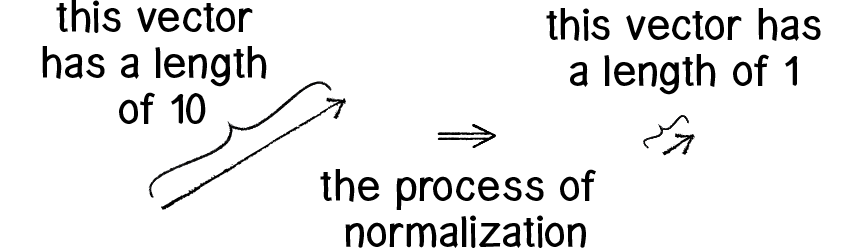A vector can be normalized by assuming a standard vector with a length of 1 and pointing it in the same direction as the original one.

This normalized vector can then be called the unit vector:A Euclidean vector represents the position of a point P in a Euclidean space. Geometrically, it can be described as an arrow from the origin of the space (vector tail) to that point (vector tip). Mathematically, a vector x in an n-dimensional Euclidean space can be defined as an ordered list of n real numbers (the Cartesian coordinates of P): x = [x1, x2, …, xn]. Its magnitude or length, denoted by , is most commonly defined as its Euclidean norm (or Euclidean length)
Magnitude (mathematics) - Wikiwand